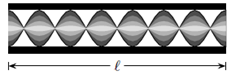# Problem: The figure below represents a sound wave in a hollow pipe with both ends open. Determine the wave length of the sound wave in this hollow pipe.1. λ = 2ℓ/92. λ = ℓ3. λ = 2ℓ/74. λ = ℓ35. λ = ℓ46. λ = ℓ27. λ = 2ℓ/38. λ = 2ℓ9. λ = 2ℓ/5

🤓 Based on our data, we think this question is relevant for Professor Del Cerro's class at HCC.

###### Problem Details

The figure below represents a sound wave in a hollow pipe with both ends open. Determine the wave length of the sound wave in this hollow pipe.

1. λ = 2ℓ/9

2. λ = ℓ

3. λ = 2ℓ/7

4. λ = ℓ3

5. λ = ℓ4

6. λ = ℓ2

7. λ = 2ℓ/3

8. λ = 2ℓ

9. λ = 2ℓ/5